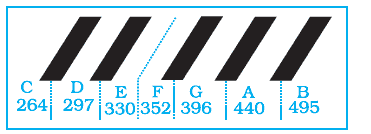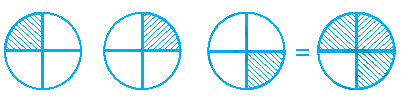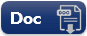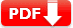# Fractions and Decimals Practice Test 2 Question Answers

Fractions and Decimals Practice Test Question Answers

Test- 2

 1. How many   \frac23 kg pieces can be cut from a cake of weight 4 kg? 🔘 A. 2 🔘 B. 6 🔘 C. 8 🔘 D. 10 🔘 e. 12
 2. Lisa had a piece of rope length of 9.5 m. She needed some small pieces of rope of length 1.9 m each. How many pieces of the required length will she get out of this rope? 🔘 A. 3 pieces 🔘 B. 5 pieces 🔘 C. 7 pieces 🔘 D. 9 pieces 🔘 E. 13 pieces
 3. Olivia uses   \frac34 m of cloth to stitch a shirt. How many shirts can she make with   2\frac14 m cloth? 🔘 A. 1 🔘 B. 2 🔘 C. 3 🔘 D. 4 🔘 E. 5
 4. If the fraction of the frequencies of two notes has a common factor between the numerator and denominator, the two notes are harmonious. Use the graphic below to find the fraction of the frequency of notes D and B.🔘 A.   \frac35 🔘 B.   \frac53 🔘 C.   \frac15 🔘 D.   \frac31 🔘 E.   \frac13
 5. Abigail said that we have gone about 120km or   \frac23 of the way to the camp site. So, how much farther do we have to go? 🔘 A. 20 km 🔘 B. 40 km 🔘 C. 60 km 🔘 D. 80 km 🔘 E. 100 km
 6.  One KG Ice cream packet requires   2\frac12  milks of flour and   1\frac23  cups of sugar. Estimated total quantity of both ingredients used in 10 such packets of Ice cream will be 🔘 A. between 10 cups and 20 cups 🔘 B. between 20 cups and 30 cups 🔘 C. between 30 cups and 40 cups 🔘 D. between 40 cups and 50 cups 🔘 E.above 50 cups
Answer: D between 40 cups and 50 cups
 7. The following diagram interprets🔘 A.   3 ÷\frac13 🔘 B.   \frac35 ÷ 3 🔘 C.   \frac13 × 4 🔘 D.   5 ÷ \frac35 🔘 E.   3 ×\frac14
 Document Type Download Link Free Editable Doc FileFree Printable PDF File